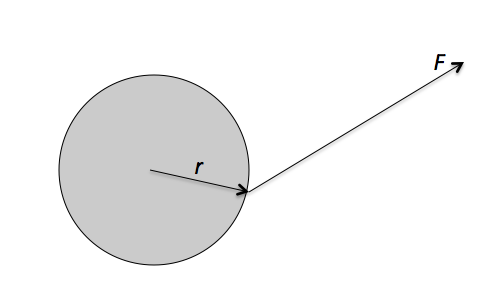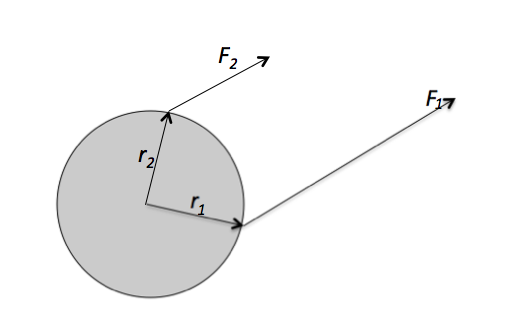Classical Mechanics

# Torque as a Cross Product

A force $$\vec{F} = \left( 7 \hat{i} + 8 \hat{k} \right) \text{ N}$$ is applied to a rotating object. If the displacement vector from the pivot to the point of action is $$\vec{r} = 3 \hat{i} \text{ m},$$ what is the torque?

Consider two vectors $$\vec{r} = 3 \hat{j} \text{ m}$$ and $$\vec{F} = \left( 6 \hat{i} + 9 \hat{k} \right) \text{ N}.$$ What is the torque in unit vector form?A force of $$\vec{F} = \left(3 \hat{i} + 6 \hat{j} \right) \text{ N}$$ is applied to a disc, as shown in the figure above. The displacement vector from the center of the disc to the force's point of action is $$\vec{r} = \left( 7 \hat{i} + 3\hat{j} \right) \text{ m}.$$ Find the torque produced by the force $$\vec{F}.$$Forces $$\vec{F_1} = \left( 6 \hat{i} + 9 \hat{j} \right) \text{ N}$$ and $$\vec{F_2} = \left( 9 \hat{i} + 14 \hat{j} \right) \text{ N}$$ are applied to a disc, as shown in the figure above. The displacement vectors from the center of the disc to the points of action of forces $$\vec{F_1}$$ and $$\vec{F_2}$$ are $$\vec{r_1} = 9 \hat{i} \text{ m}$$ and $$r_2 = 5 \hat{j} \text{ m},$$ respectively. Find the net torque produced by the forces.

A plum is located at $$( 9 \text{ m}, 12 \text{ m} )$$ on the $$xy$$-coordinate plane. If a force of $$\vec{F} = ( 4 \text{ N} , 8 \text{ N} )$$ is applied to the plum, what is the torque relative to the origin $$O?$$

×